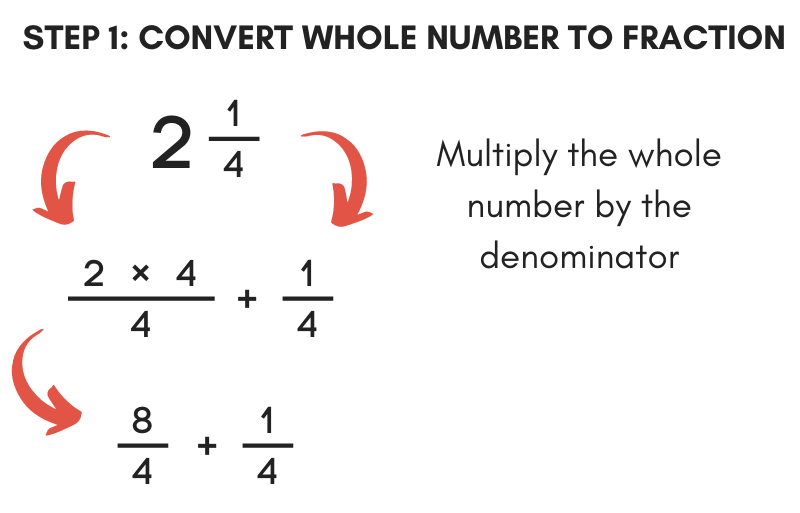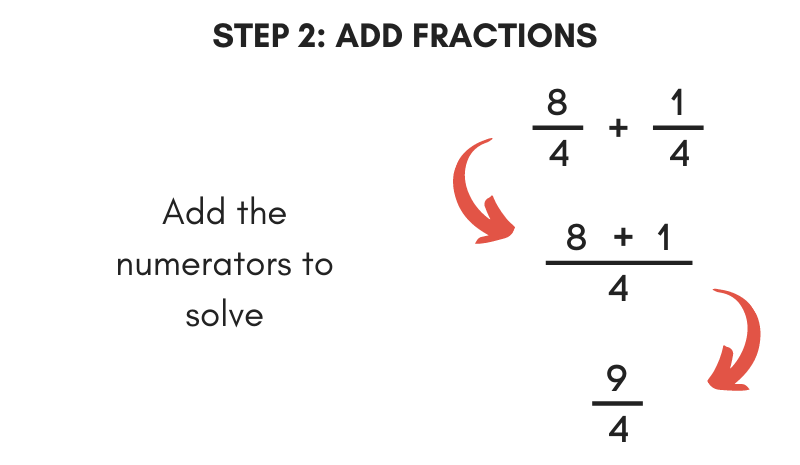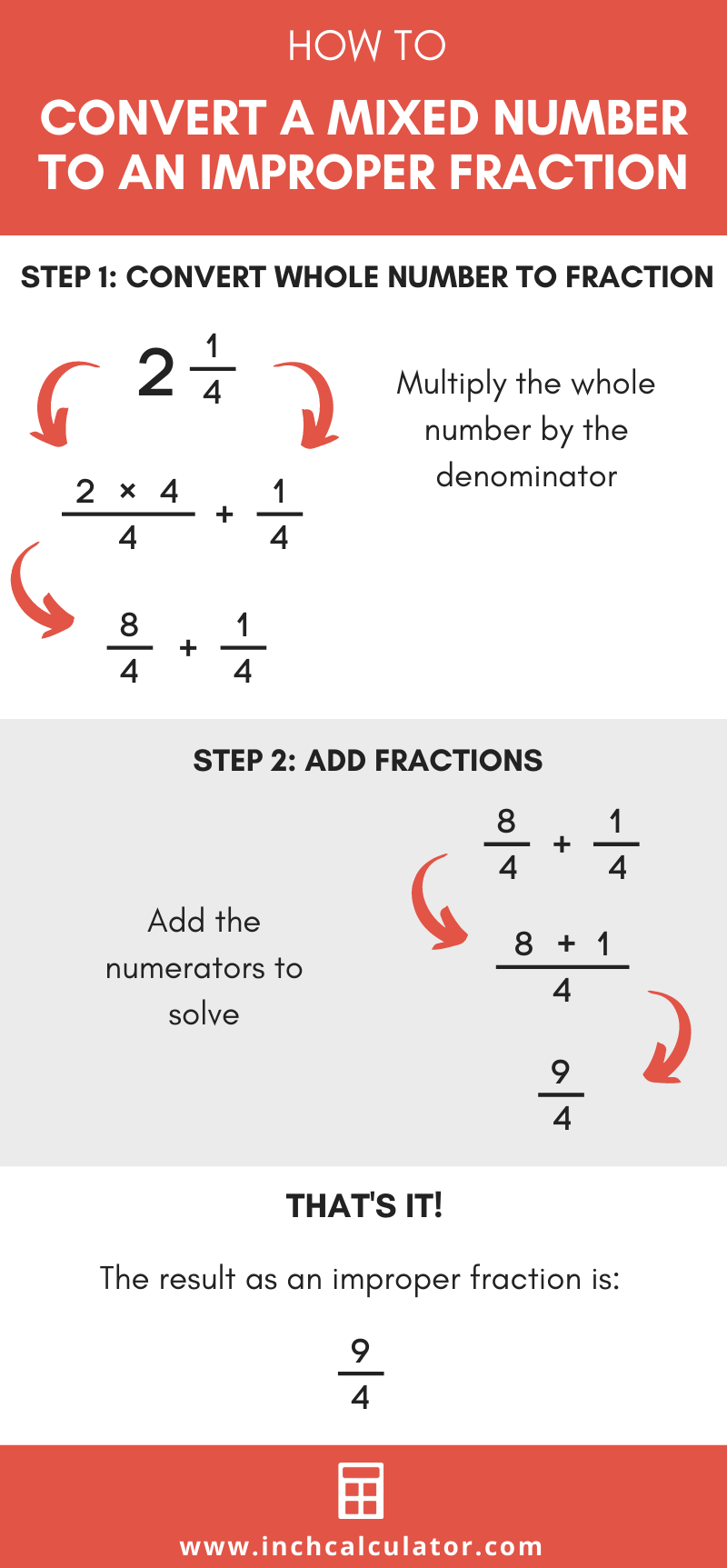# Mixed Number to Improper Fraction Calculator

Convert a mixed number to an improper fraction using the calculator below. Follow along to see each step to make the conversion yourself.

Fraction:

## Improper Fraction Result:

7 / 3

### Steps to Convert Mixed Fraction to Improper Fraction

Convert the whole number to a fraction by multiplying the whole number by the denominator
2=2 × 3 / 3
2=6 / 3
Add the converted whole number to the remainder from the mixed number to solve the improper fraction
6 + 1 / 3
7 / 3
Learn how we calculated this below

## How to Convert a Mixed Number to an Improper Fraction

A mixed number is a number represented as using a whole number and a fraction. A mixed number is a fraction that, when reduced, has a numerator larger than the denominator.

Follow these two easy steps to convert a mixed number to an improper fraction.

### Step One: Convert the Whole Number to a Fraction

The first step to converting a mixed number to a fraction is to convert the whole number to a fraction. To do this, multiply the whole number by the denominator of the fraction.

Then put the result over the denominator.For example, let’s convert the whole number 2 in the mixed fraction 2 1 / 4 to a fraction.

First, let’s find the numerator:
numerator = 2 × 4
numerator = 8

Then put the numerator over the original denominator 4:
fraction = 8 / 4

### Step Two: Add the Fractions to Solve the Improper Fraction

The next step is to add the fraction from the last step with the fraction in the mixed number to find the improper fraction.

To do this, add the numerators of each fraction together, then put the sum over the denominator. The result is an improper fraction.For example, add 8 / 4 and 1 / 4 to find the fraction.